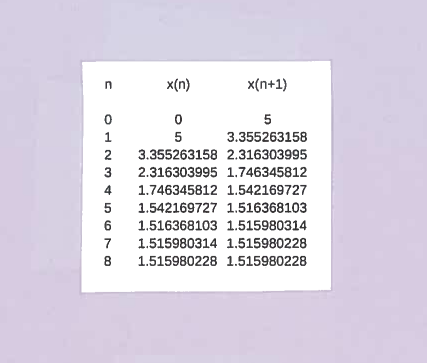SOLUTION 1: We are given the equation $x^3+x-5=0$, so let's define function $f(x) = x^3+x-5$, whose graph is given below.The derivative of $f$ is $f'(x) = 3x^2+1$. Now use Newton's Method: $$x_{n+1} = x_{n} - { f(x_{n}) \over f'(x_{n}) } \ \ \ \ \longrightarrow$$ $$x_{n+1} = x_{n} - { x_{n}^3+x_{n}-5 \over 3x_{n}^2+1} \ \ \ \ \longrightarrow$$ (Let's simplify the right-hand side of this equation. First get a common denominator.) $$x_{n+1} = x_{n} \ { 3x_{n}^2+1 \over 3x_{n}^2+1 } - { x_{n}^3+x_{n}-5 \over 3x_{n}^2+1} \ \ \ \ \longrightarrow$$ $$x_{n+1} = { 3x_{n}^3+ x_{n} \over 3x_{n}^2+1 } - { x_{n}^3+x_{n}-5 \over 3x_{n}^2+1} \ \ \ \ \longrightarrow$$ $$x_{n+1} = { 3x_{n}^3+ x_{n} - ( x_{n}^3+x_{n}-5 ) \over 3x_{n}^2+1 } \ \ \ \ \longrightarrow$$ $$x_{n+1} = { 2x_{n}^3+5 \over 3x_{n}^2+1 }$$ a.) Let $x_{0}=0$. Then using Newton's Method formula we get that $$x_{1} = { 2x_{0}^3+5 \over 3x_{0}^2+1 } = { 2(0)^3+5 \over 3(0)^2+1 } = 5$$ and $$x_{2} = { 2x_{1}^3+5 \over 3x_{1}^2+1 } = { 2(5)^3+5 \over 3(5)^2+1 } = { 255 \over 76 } \approx 3.355263158$$ Using Newton's Method formula for 8 iterations in a spreadsheet results in :Thus the solution $r$ to the original equation to five decimal places is $r \approx 1.51598$.

b.) Let $x_{0}=1$. Then using Newton's Method formula we get that $$x_{1} = { 2x_{0}^3+5 \over 3x_{0}^2+1 } = { 2(1)^3+5 \over 3(1)^2+1 } = { 7 \over 4} = 1.75$$ and $$x_{2} = { 2x_{1}^3+5 \over 3x_{1}^2+1 } = { 2(1.75)^3+5 \over 3(1.75)^2+1 } = { 1006 \over 652 } \approx 1.54294$$ Using Newton's Method formula for 5 iterations in a spreadsheet results in :Thus the solution $r$ to the original equation to five decimal places is $r \approx 1.51598$.

c.) Let $x_{0}=-1$. Then using Newton's Method formula we get that $$x_{1} = { 2x_{0}^3+5 \over 3x_{0}^2+1 } = { 2(-1)^3+5 \over 3(-1)^2+1 } = { 3 \over 4 } =0.75$$ and $$x_{2} = { 2x_{1}^3+5 \over 3x_{1}^2+1 } = { 2(0.75)^3+5 \over 3(0.75)^2+1 } = { 374 \over 172 } \approx 2.17441$$ Using Newton's Method formula for 7 iterations in a spreadsheet results in :Thus the solution $r$ to the original equation to five decimal places is $r \approx 1.51598$.